# ML Aggarwal Class 6 Solutions for ICSE Maths Chapter 7 Decimals Objective Type Questions

## ML Aggarwal Class 6 Solutions for ICSE Maths Chapter 7 Decimals Objective Type Questions

Mental Maths
Question 1.
Fill in the blanks:
(i) The decimal point in a decimal number is placed between ones digit and ……….. digit.
(ii) The place value of the digit 3 in the decimal number 15.437 is ………
(iii) The decimal number 27.025 has ………. decimal places.
(iv) The decimal number 5.06 is read as five point
(v) If an object is divided into 1000 equal parts, then its 27 parts are represented by ………
(vi) Two decimal numbers having different number of …………. are called unlike decimal numbers.
(vii) 4 tens, 3 ones, 2 tenths, 0 hundredths and 5 thousandths in decimal form is written as ……
(viii)The smallest decimal number upto three decimal places is ……….
Solution: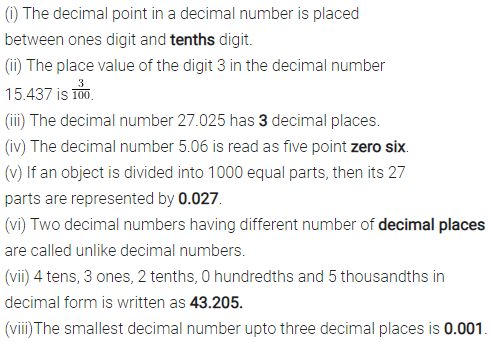Question 2.
State whether the following statements are true (T) or false (F):
(i) Every decimal number can be represented by a point on a number line.
(ii) Fractions with denominator 10, 100, 1000, are called decimal fractions.
(iii) A decimal number having 3 decimal places can be written as a fraction with denominator 1000.
(iv) The value of a decimal number remains the same if any number of extra zeros are written at the end of a decimal number.
(v) If a decimal number is multiplied by 10, then the decimal point moves by one place to the left.
Solution: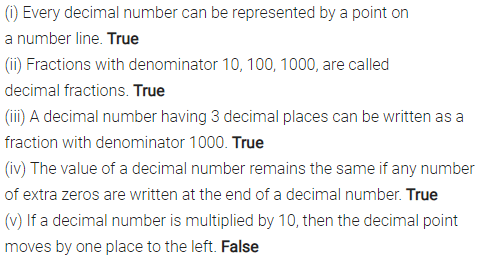Multiple Choice Questions
Choose the correct answer from the given four options (3 to 23):
Question 3.
Five and seven hundredths is equal to
(a) 5.7
(b) 5.07
(c) 5.70
(d) 0.57
Solution:Question 4.
Sicty three thousandths is equal to
(a) 0.63
(b) 0.603
(c) 0.063
(d) 0.630
Solution:Question 5.
$$3 \frac{7}{100}$$ is equal to :
(a) 3.07
(b) 3.7
(c) 3.70
(d) 3.007
Solution:Question 6.
$$5 \frac{3}{1000}$$ is equal to
(a) 5.03
(b) 5.3
(c) 5.003
(d) 5.0003
Solution: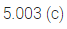Question 7.
The place value of the digit 7 in the decimal number 5.0378 is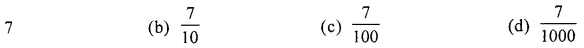Solution: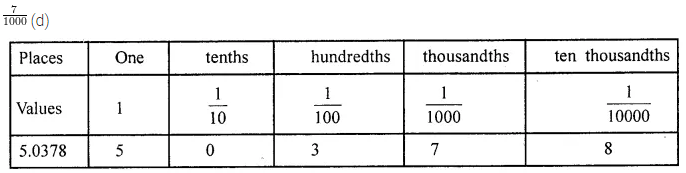Question 8.
The place value of the digit 0 in the decimal number 13.405 is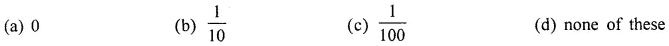Solution: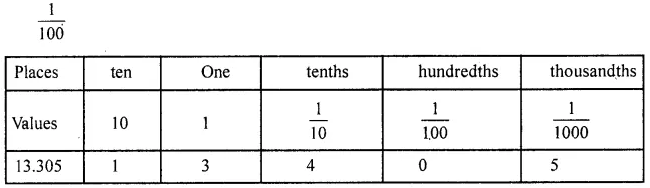Question 9.
The value of $$5+\frac{7}{10}+\frac{3}{1000}$$ is
(a) 5.73
(b) 5.703
(c) 5.073
(d) 0.753
Solution:Question 10.
The value of $$\frac{3}{25}$$ is
(a) 1.2
(b) 0.012
(c) 0.12
(d) none of these
Solution: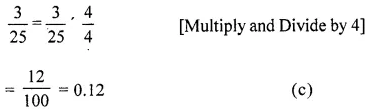Question 11.
The value of $$5 \frac{1}{25}$$ is
(a) 5.4
(b) 5.25
(c) 5.04
(d) 5.004
Solution:Question 12.
The decimal number not equivalent to 5.7 is
(a) 5.70
(b) 5.07
(c) 5.700
(d) 5.7000
Solution: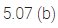Question 13.
1 g is equal to
(a) 0.1 kg
(b) 0.01 kg
(c) 0.001 kg
(d) 0.0001 kg
Solution: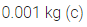Question 14.
2 km 7 m is equal to
(a) 2.7 km
(b) 2.07 km
(c) 2.007 km
(d) 2.0007 km
Solution: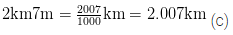Question 15.
Among 2.34, 2 .43, 2.344 and 2.4, the greatest number is
(a) 2.34
(b) 2.43
(c) 2.344
(d) 2.4
Solution: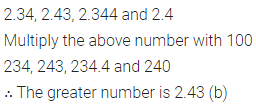Question 16.
5.2 – 3.6 is equal to
(a) 0.16
(b) 2.6
(c) 0.26
(d) 1.6
Solution:Question 17.
A decimal number lying between 2.2 and 2.22 is
(a) 2.12
(b) 2.23
(c) 2.219
(d) 2.3
Solution: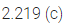Question 18.
0.023 lies between
(a) 0.2 and 0.3
(b) 0.02 and 0.03
(c) 0.029 and 0.03
(d) 0.026 and 0.024
Solution:Question 19.
0.7499 lies between
(a) 0.7 and 0.74
(b) 0.759 and 0.799
(c) 0.749 and 0.75
(d) 0.74992 and 0.75
Solution: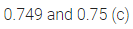Question 20.
Which of the following decimal numbers is the greatest?
(a) 0.182
(b) 0.038
(c) 0.219
(d) 0.291
Solution: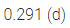Question 21.
Which of the following decimal numbers is the smallest?
(a) 0.108
(b) 1.08
(c) 0.801
(d) 0.81
Solution: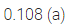Question 22.
0.003 × 0.2 is equal to
(a) 0.6
(b) 0.06
(c) 0.006
(d) 0.0006
Solution:Question 23.
0.45 + 0.9 is equal to
(a) 50
(b) 5
(c) 0.5
(d) 0.05
Solution: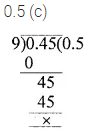Higher Order Thinking Skills(Hots)
Question 1.
On her birthday, Ayushi is taking her 5 friends to a movie and treats them with cold costs. The cost of a ticket is ₹150 and a cold drink cost ₹28.50. How much Ayushi has to spend?
Solution: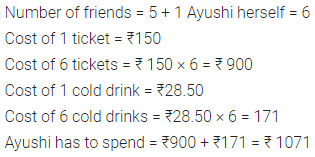Question 2.
Write digits in the boxes of the number : $$3 \square 6 \square .8 \square \square$$ to obtain
(i) greatest possible number
(ii) smallest possible number. Repetition of digits in a number is not allowed.
Solution: